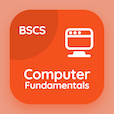Engineering Courses Online

Control Systems Prep Tests

# Questions on Feedback Control System with Answers PDF

The e-Book Questions on Feedback Control System with Answers Multiple Choice Questions (MCQ Quiz), Control Systems quiz answers PDF to study online courses, control systems tests. Solve Phase Margin Multiple Choice Questions and Answers (MCQs), Control Systems quiz questions to enroll in online classes. The e-Book "Questions on Feedback Control System with Answers MCQ" App Download: pid controller objective exam questions with answers, control system interview questions for freshers, control systems comprehensive viva questions with answers, control system problems, formulas and solutions test prep for undergraduate engineering schools.

The MCQ "Amount of additional open loop phase shift required at unity gain to make the closed loop system unstable is called" PDF, Questions on Feedback Control System with Answers App Download (Free) with gain margin, phase margin, amplitude margin, and frequency margin choices to enroll in online classes. Practice questions on feedback control system with answers quiz questions, download Google eBook (Free Sample) for online engineering associate's degree.

## MCQs: Questions on Feedback Control System with Answers

MCQ: Amount of additional open loop phase shift required at unity gain to make the closed loop system unstable is called

A) gain margin
B) phase margin
C) amplitude margin
D) frequency margin

MCQ: Frequency at which magnitude frequency response plots equal to is 0dB is called

A) gain margin frequency
B) natural frequency
C) phase margin frequency
D) phase margin frequency

MCQ: State variables such that each subsequent state variable is the derivative of the previous state variable is called

A) phase variable
B) gain variable
C) amplitude variable
D) frequency variable

MCQ: Phasor is also known as

A) complexor
B) phase
C) gain
D) Nyquist phase

MCQ: Complex number which represents a sinusoidal function whose amplitude, angular frequency, and initial phase are time-invariant is called

A) phase
B) gain
C) phasor
D) Nyquist phase

### Practice Tests: Control Systems Exam Prep

Download Basic Control Systems Quiz App, General Knowledge MCQs App and ICT MCQ App for Android & iOS devices. These Apps include complete analytics of real time attempts with interactive assessments. Download Play Store & App Store Apps & Enjoy 100% functionality with subscriptions!